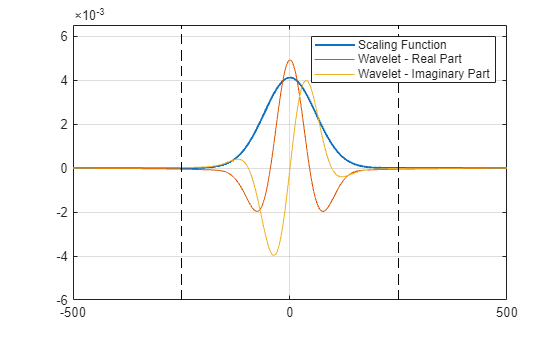# Wavelet Scattering Invariance Scale and Oversampling

This example shows how changing the invariance scale and oversampling factor affects the output of the wavelet scattering transform.

### Invariance Scale

The `InvarianceScale` property of a wavelet time scattering network sets the time scale of the scaling (lowpass) filter. Create a wavelet scattering network with a signal length of 10,000 and invariance scale of 500. Obtain the filter bank.

```sigLength = 1e4; sf = waveletScattering('SignalLength',sigLength,'InvarianceScale',500); [fb,f] = filterbank(sf);```

Use the helper function `helperPlotScalingWavelet` to plot the scaling filter in time along with the real and imaginary parts of the coarsest-scale wavelet from the first filter bank. The source code for `helperPlotScalingWavelet` is listed in the appendix. The supports of the scaling filter and wavelet are essentially the size of the invariance scale.

`helperPlotScalingWavelet(fb,f,500)`Generate a random signal and use `featureMatrix` to obtain the scattering feature matrix for the signal and scattering network.

```x = randn(1,sigLength); smat = featureMatrix(sf,x); whos smat```
``` Name Size Bytes Class Attributes smat 102x79 64464 double ```

Each row of the feature matrix is a vector which has been convolved (filtered) with the lowpass filter (after other wavelet filtering). The second dimension of the feature matrix is the time resolution. The output of the filtering is downsampled as much as possible without aliasing, what is called "critically downsampling". The amount of downsampling depends on the bandwidth of the filter. The bigger the invariance scale, the larger the time support of the lowpass (scaling) function and accordingly the more we can downsample.

Obtain the scattering transform of the signal.

```[S,~] = scatteringTransform(sf,x); S{2}```
```ans=41×4 table signals path bandwidth resolution _____________ _______ _________ __________ {79x1 double} 0 1 0.0084478 -7 {79x1 double} 0 2 0.0084478 -7 {79x1 double} 0 3 0.0084478 -7 {79x1 double} 0 4 0.0084478 -7 {79x1 double} 0 5 0.0084478 -7 {79x1 double} 0 6 0.0084478 -7 {79x1 double} 0 7 0.0084478 -7 {79x1 double} 0 8 0.0084478 -7 {79x1 double} 0 9 0.0084478 -7 {79x1 double} 0 10 0.0084478 -7 {79x1 double} 0 11 0.0084478 -7 {79x1 double} 0 12 0.0084478 -7 {79x1 double} 0 13 0.0084478 -7 {79x1 double} 0 14 0.0084478 -7 {79x1 double} 0 15 0.0084478 -7 {79x1 double} 0 16 0.0084478 -7 ⋮ ```

The scattering coefficient vectors, `signals`, have length 79, and the resolution is -7. This means that we expect approximately $1{0}^{4}/{2}^{7}$ coefficients in each vector.

Create a wavelet scattering network with an invariance scale of 200. Obtain the scattering transform of the signal. Because the invariance scale is smaller, the bandwidth of the scaling filter increases and we cannot downsample as much without aliasing. Therefore, the number of scattering coefficients increases.

```sf = waveletScattering('SignalLength',sigLength,'InvarianceScale',200); [S,~] = scatteringTransform(sf,x); S{2}```
```ans=30×4 table signals path bandwidth resolution ______________ _______ _________ __________ {157x1 double} 0 1 0.02112 -6 {157x1 double} 0 2 0.02112 -6 {157x1 double} 0 3 0.02112 -6 {157x1 double} 0 4 0.02112 -6 {157x1 double} 0 5 0.02112 -6 {157x1 double} 0 6 0.02112 -6 {157x1 double} 0 7 0.02112 -6 {157x1 double} 0 8 0.02112 -6 {157x1 double} 0 9 0.02112 -6 {157x1 double} 0 10 0.02112 -6 {157x1 double} 0 11 0.02112 -6 {157x1 double} 0 12 0.02112 -6 {157x1 double} 0 13 0.02112 -6 {157x1 double} 0 14 0.02112 -6 {157x1 double} 0 15 0.02112 -6 {157x1 double} 0 16 0.02112 -6 ⋮ ```

### Oversampling Factor

Because the invariance scale is such an important hyperparameter for scattering networks (one of the most important for performance), you should set the value based on the problem at hand and not because you want a certain number of coefficients. You can use the `OversamplingFactor` property for adjusting the number of coefficients for a given `InvarianceScale`. The `OversamplingFactor` specifies how much the scattering coefficients are oversampled with respect to the critically downsampled values. The factor is on a ${\mathrm{log}}_{2}$ scale.

Create a scattering network with an invariance scale of 500, and an `OversamplingFactor` equal to 1. Obtain the scattering transform of the signal. As expected, the number of scattering paths is greater than in the case where `InvarianceScale` is 200. By setting `OversamplingFactor` to 1, the scattering transform returns two times as many coefficients for each scattering path with respect to the critically sampled number. The size of the scattering coefficient vectors returned is equal to the size when the scattering network has an invariance scale of 200 with default critical downsampling.

```sf = waveletScattering('SignalLength',sigLength,'InvarianceScale',500,'OversamplingFactor',1); [S,~] = scatteringTransform(sf,x); S{2}```
```ans=41×4 table signals path bandwidth resolution ______________ _______ _________ __________ {157x1 double} 0 1 0.0084478 -6 {157x1 double} 0 2 0.0084478 -6 {157x1 double} 0 3 0.0084478 -6 {157x1 double} 0 4 0.0084478 -6 {157x1 double} 0 5 0.0084478 -6 {157x1 double} 0 6 0.0084478 -6 {157x1 double} 0 7 0.0084478 -6 {157x1 double} 0 8 0.0084478 -6 {157x1 double} 0 9 0.0084478 -6 {157x1 double} 0 10 0.0084478 -6 {157x1 double} 0 11 0.0084478 -6 {157x1 double} 0 12 0.0084478 -6 {157x1 double} 0 13 0.0084478 -6 {157x1 double} 0 14 0.0084478 -6 {157x1 double} 0 15 0.0084478 -6 {157x1 double} 0 16 0.0084478 -6 ⋮ ```

### Appendix

```function helperPlotScalingWavelet(fb,f,invScale) % This function is in support of wavelet scattering examples only. It may % change or be removed in a future release. fBin = diff(f(1:2)); time = (-1/2:fBin:1/2-fBin)*1e4; phi = ifftshift(ifft(fb{1}.phift)); psiL1 = ifftshift(ifft(fb{2}.psift(:,end))); figure plot(time,phi,'b','LineWidth',1.5) grid on hold on plot(time,real(psiL1)); plot(time,imag(psiL1)); plot([-invScale/2 -invScale/2],[-6e-3 6.5e-3],'k--') plot([invScale/2 invScale/2],[-6e-3 6.5e-3],'k--') ylim([-6e-3 6.5e-3]) xlim([-invScale invScale]) legend('Scaling Function','Wavelet-Real Part','Wavelet-Imaginary Part') end```